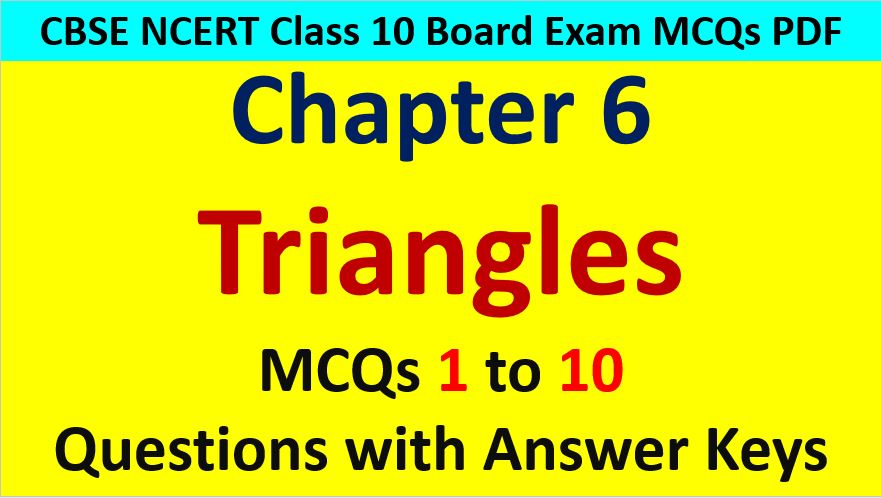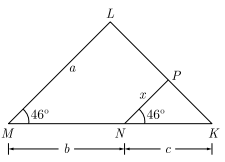Friday, October 22, 2021
Home > CBSE Class 10 > MCQ Questions for Class 10 Maths Chapter 6 Triangles with Answer Keys

# MCQ Questions for Class 10 Maths Chapter 6 Triangles with Answer KeysHi students, Welcome to AMBiPi (Amans Maths Blogs). In this article, you will get MCQ Questions for Class 10 Maths Chapter 6 Triangles with Answer Keys PDF. You can download this PDF and save it in your mobile device or laptop etc.

MCQ Questions for Class 10 Maths Chapter 6 Triangles Question No 1:

If in triangles ABC and DEF, AB/EF = AC/DE, then the triangles will be similar if

Option A : ∠A = ∠D

Option B : ∠A = ∠E

Option C : ∠B = ∠E

Option D : ∠C = ∠F

Option B : A = E

MCQ Questions for Class 10 Maths Chapter 6 Triangles Question No 2:

In the given figure, DE || BC. The value of EC isOption A : 1.5 cm

Option B : 3 cm

Option C : 2 cm

Option D : 1 cm

Option C : 2 cm

MCQ Questions for Class 10 Maths Chapter 6 Triangles Question No 3:

A square and a rhombus are always

Option A : similar

Option B : congruent

Option C : similar but not congruent

Option D : neither similar nor congruent

Option D : neither similar nor congruent

MCQ Questions for Class 10 Maths Chapter 6 Triangles Question No 4:

In the given figure, x isOption A : ab/(a + b)

Option B : ac/(b + c)

Option C : bc/(b + c)

Option D : ac/(a + c)

Option B : ac/(b + c)

MCQ Questions for Class 10 Maths Chapter 6 Triangles Question No 5:

If ΔABC ~ ΔDEF and EF = BC/3, then then ar(ΔABC):(ΔDEF) is

Option A : 3 : 1

Option B : 1 : 3

Option C : 1 : 9

Option D : 9 : 1

Option C : 1 : 9

MCQ Questions for Class 10 Maths Chapter 6 Triangles Question No 6:

Triangle ABC is an equilateral triangle with each side of length 2p. If AD is perpendicular to BC then the value of AD is

Option A : √3

Option B : √3p

Option C : 2p

Option D : 4p

Option B : √3p

MCQ Questions for Class 10 Maths Chapter 6 Triangles Question No 7:

If a triangle and a parallelogram are on the same base and between same parallels, then what is the ratio of the area of the triangle to the area of parallelogram?

Option A : 1 : 2

Option B : 3 : 2

Option C : 1 : 3

Option D : 4 : 1

Option A : 1 : 2

MCQ Questions for Class 10 Maths Chapter 6 Triangles Question No 8:

Which of the following statement is false?

Option A : All isosceles triangles are similar

Option B : All quadrilateral are similar.

Option C : All circles are similar

Option D : None of the above

Option A : All isosceles tringles are similar

MCQ Questions for Class 10 Maths Chapter 6 Triangles Question No 9:

D and E are respectively the points on the sides AB and AC of a triangle ABC such that AD = 2 cm, BD = 3 cm, BC = 7.5 cm and DE || BC. Then, length of DE (in cm) is

Option A : 2.5

Option B : 3

Option C : 5

Option D : 6

Option B : 3

MCQ Questions for Class 10 Maths Chapter 6 Triangles Question No 10:

Two poles of height 6 m and 11 m stand vertically upright on a plane ground. If the distance between their foot is 12 m, then distance between their tops is

Option A : 12 cm

Option B : 14 cm

Option C : 13 cm

Option D : 11 cm